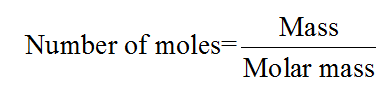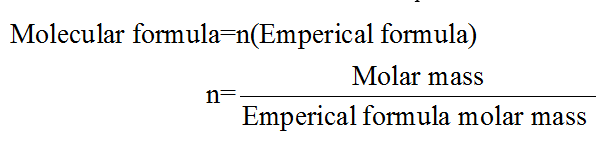# A compound is 19.3% sodium, 26.9% sulfur, and 53.8% oxygen. Its formula mass is 238.00 g. What is the empirical formula?Name the compound and calculate its molar mass.

Question
88 views
A compound is 19.3% sodium, 26.9% sulfur, and 53.8% oxygen. Its formula mass is 238.00 g. What is the empirical formula?
Name the compound and calculate its molar mass.
check_circle

Step 1

The number of moles for a given chemical species refers to the ratio of mass of the species to that of its molar mass. The formula is shown below:Step 2

The empirical formula for a given chemical compound is used to describe the simplest whole number ratio of all the atoms that are present in it.

The relation between molecular formula and empirical formula is as follows:Step 3

In the given problem, it has been given that, given compound contains 19.3% of Sodium, 26.9% of Sulphur and 53.8% of oxygen.

If it is assumed that the mass of the given compound is 100 g, then it will contain 19.3 g of sodium, 26.9 g of Sulphur and 53.8 g of oxygen. The number of moles of each of the elem...

### Want to see the full answer?

See Solution

#### Want to see this answer and more?

Solutions are written by subject experts who are available 24/7. Questions are typically answered within 1 hour.*

See Solution
*Response times may vary by subject and question.
Tagged in